3.1. Program Graph Cleaning

Now I will show how to make one more - and maybe the most promising - metasystem transition in program transformation [40,45]. As discussed in Sec.2.1, one step of the Refal machine is represented in the pattern-matching graph as a normal walk C1R1A1. For driving we combine two steps by concatenating two normal walks: C1R1A1C2R2A2, and normalize them into one walk again. We can postpone driving and combine any number of elementary walks into unnormalized walks of arbitrary length. Such walks will represent possible (but not necessarily feasible) histories of computation without performing the computation itself. Take the graph

  ([]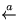y)   (x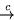s.1 x) { (s.1'a')(y'b'y)  +(# s.1'a') (y s.1y)  } +(x[]) []out

from Sec.2.1. Denote the one-step walks in the graph as follows:

 w1 = ([]y) w2 = (xs.1 x) (s.1'a') (y'b'y) w3 = (xs.1 x) (# s.1'a') (y s.1y) w4 = (x[]) []out

Now the set of all terminated walks (histories of completed computation) is described by the regular grammar: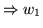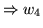or by the regular expression w(w+w)*w . It is easy to see that for an arbitrary Refal program the set of all walks is defined by a context-free grammar, while if we restrict ourselves to flat Refal (no nested calls) the walk grammar becomes regular, hence the whole set is represented by a regular expression, which is a great advantage of the flat version. We shall work with a generalization of regular expressions where the number of iterations is denoted by a variable, so that such walk-sets as wnwn are permitted.

Walk-sets are an alternative form of a program. We can define an interpreter Int which executes such programs, and transform Int calls by the supercompiler: an MST to the three-level hierarchy: Scp-Int-Program. But what do we achieve by this MST? Well, if Int does just driving, i.e., processes the walks in the strict order from left to right, we have achieved nothing. But we can create a clever interpreter which uses equivalence relations on the set of walks. It could unwind iterative loops both from the left, and from the right, i.e., use the relation Wn+1 = W Wn or Wn+1 = WnW in order to achieve reduction in supercompilation. It could also reorder operations in walks using commutation relations and do other transformations. In  I show that with this technique we can make transformations which could not be done by direct supercompilation, such as function inversion and the merging of consecutive iterative loops.

The Scp-Int technique can be very effectively used for graph cleaning. Suppose we finished supercompilation and have the resulting flat graph G. It does not mean that all exit nodes (expressions for output) are actually feasible. For each node in G we can write a regular expression for the set of all walks which lead to this node. Using the Scp-Int method we may discover that some walk-sets are reduced to Z; then these nodes can be eliminated.

As an example, let us return to the proof of commutativity of addition, which we have found more difficult to prove than the other theorems in Sec.2.3. The proof requires a considerable analysis of the graph resulting from straightforward supercompilation. I will only sketch the proof.

The configuration to compute is

 <= <+ x,y>, <+ y,x>>

In the process of supercompilation,  is generalized, and reduced to the generalized configuration:

 ( x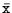)(y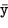)  <= <+,y>, <+,x>>

The graph for  has 15 exits. Four of them are T, eleven are F. To prove the theorem, the walks which lead to each of the eleven F ends must be proven unfeasible. As an example, I will do it for one of the walks, which is neither the least nor the most difficult:

 w= (x)(y)(yy'1')n(xx'1')n(yy'1')(x'0') ('1')('1')m(yy'1')m('0')(yy'1')

Here m and n are variables, so w represents an infinite set of walks, not a single walk.

Using commutation relations we reduce w to the form:

 w= (x)(xx'1')n(x'0') (y)('1')p('0')(yy'1')q

where we have introduced new variables p,q, which show the total number of iterations in the walk. Their relation to the loop variables m,n, namely p=m+1 and q=n+m+2, will be treated as a restriction on p,q. The unfeasibility of w results from the second line, i.e., y-part of it; so we ignore the first line (x-part). The call of the interpreter takes the form:This is a function of p and q. Unwinding the p-loop means breaking it into a recursive call and the base: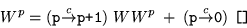and analogously for the q-loop.

Thus, driving produces branches to four cases:

    (pp+1)(qq+1)  + (pp+1)(q'0')  + (p'0')(qq+1)  + (p'0')(q'0') 

Let us consider the walk transformation in . This is the case when recursion takes place in both p-loop, and q-loop:

(y)('1')('1')p('0')(yy'1')(yy'1')q

Resolving the clash on, we have:

(y)('1') = ( Y :'1') = (yy'1')(y)(y'1'y)

Now the assignment (y'1'y) travels to the right and clashes with contraction (yy'1') to produce nothing. The contraction (yy'1') is taken from the argument of Int and becomes a contraction on the input variable y (I cannot go here into formal details). The result is that  becomes identical to : a reduction (folding).

It is easy to check, that configurations  and  are unfeasible. Configuration  is an exit from the loop where p and q are decreased by one in every cycle. If we denote the number of cycles as N, the restriction on p,q becomes:

p  +  N  = m + 1,  q +  N  =  n + m + 2

When p=q=0, we have the restriction m+1=n+m+2, which cannot be satisfied because  n > 0. The graph for  becomes a loop without exits:

 <Int w> (pp+1) (qq+1) 

This completes the proof of unfeasibility of the chosen walk. The other ten walks are handled analogously.

Contents  Next